# bar3h

Horizontal 3-D bar graph

•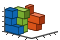## Syntax

``bar3h(y)``
``bar3h(z,y)``
``bar3h(___,width)``
``bar3h(___,style)``
``bar3h(___,color)``
``bar3h(ax,___)``
``h = bar3h(___)``

## Description

example

````bar3h(y)` creates a horizontal 3-D bar graph for the elements of `y`. Each bar corresponds to an element in `y`. To plot a single series of bars, specify `y` as a vector. For a vector of length m, the function plots the bars on a z-axis ranging from `1` to m.To plot multiple series of bars, specify `y` as a matrix with one column for each series. For an m-by-n matrix, the function plots the bars on an x-axis ranging from `1` to n and a z-axis ranging from `1` to m. ```

example

````bar3h(z,y)` creates a horizontal bar graph of the elements in `y` at the z-values specified in `z`.```

example

````bar3h(___,width)` sets the width of the bars along the x- and z-axes and controls the separation of bars within a group. By default, `width` is `0.8` and the bars have a slight separation. If `width` is `1`, then the bars within a group do not have any space between them. Specify `width` in addition to any of the input argument combinations in previous syntaxes.```

example

````bar3h(___,style)` specifies the style of the bars, where `style` can be `"detached"`, `"grouped"`, or `"stacked"`. The default style is `"detached"`.```

example

````bar3h(___,color)` displays all bars using the color specified by `color`. For example, use `"r"` to specify all red bars.```

example

````bar3h(ax,___)` plots into the axes specified by `ax` instead of into the current axes (`gca`). The option `ax` can precede any of the input argument combinations in the previous syntaxes.```

example

````h = bar3h(___)` returns one or more `Surface` objects. If `y` is a vector, then `bar3h` creates one `Surface` object. If `y` is a matrix, then `bar3h` returns a `Surface` object for each series. Use `h` to set properties of the bars after displaying the bar graph. For a list of properties, see Surface Properties.```

## Examples

collapse all

Specify `y` as a vector of five values. Plot these values as a series of horizontal 3-D bars, with the height of each bar corresponding to a value in `y` and its z-axis location corresponding to the index of that value.

```y = [50 40 30 20 10]; bar3h(y);```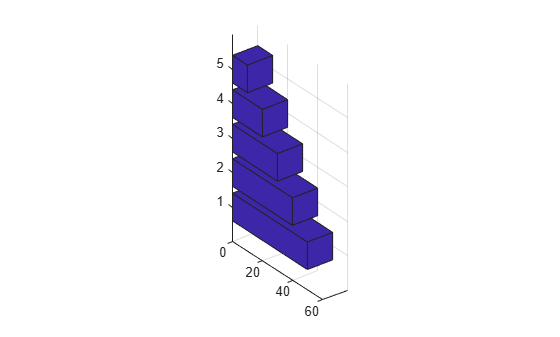Specify `y` as a matrix. Create a horizontal 3-D bar graph of `y` with each series corresponding to a column in `y`.

```y = [1 4 7; 2 5 8; 3 6 9; 4 7 10]; bar3h(y)```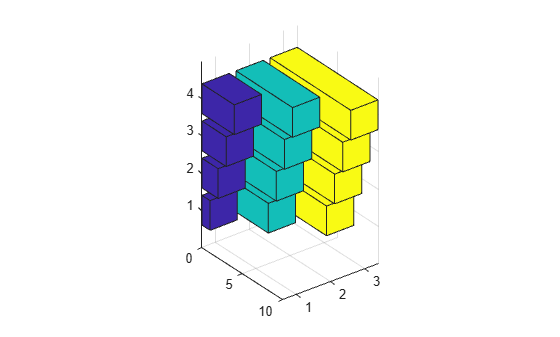Specify `z` as a vector of z-axis locations for the bars in `y`. Plot the bars at the specified z-axis locations.

```z = [1950 1960 1970 1980 1990]; y = [16 8 4 2 1]; bar3h(z,y)```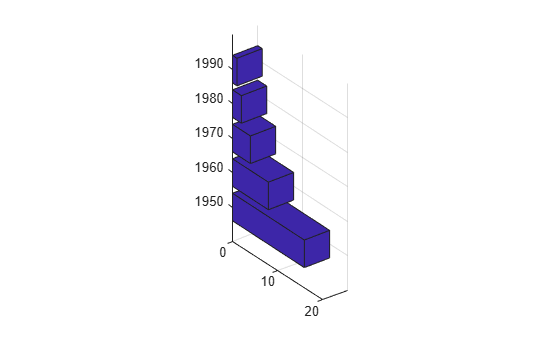Specify `y` as a matrix with four series. Plot `y` with the data for each row grouped together by using the `'grouped'` style. To eliminate space between bars of the same group, set `width` to `1`.

```y = [70 50 33 10; 75 55 35 15; 80 60 40 20]; bar3h(y,1,'grouped')```Create a set of z-coordinates ranging from `0` to π. Plot sine functions of the z-values as a horizontal 3-D bar graph. Use the color specification `"r"` to make the bars red.

```z = 0:pi/16:pi; y = [sin(z')/4 sin(z')/2 sin(z')]; bar3h(z,y,1,"r")```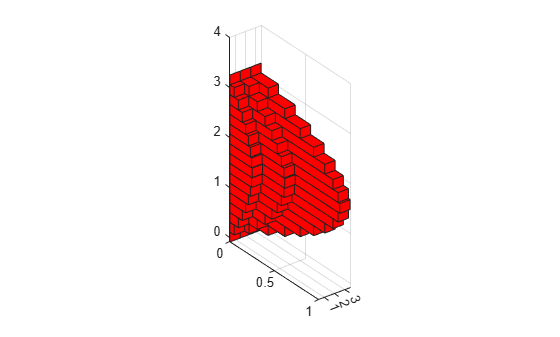Plot data matrix `y` with the bar style `"stacked"` and bar width `0.5`. Store the returned `Surface` objects in `h`. In this case, `h` has four elements, one for each column in `y`.

Note that `bar3h` colors each series based on the default colormap.

```y = [19 30 21 30; 40 16 32 12]; h = bar3h(y,0.5,"stacked");```Customize the color of each series by setting the `FaceColor` of the corresponding object in `h`.

```h(1).FaceColor = "k"; h(2).FaceColor = "white"; h(3).FaceColor = [.5 .7 .8]; h(4).FaceColor = [.9 .7 .8];```Since R2019b

You can display a tiling of plots using the `tiledlayout` and `nexttile` functions. Call the `tiledlayout` function to create a 1-by-2 tiled chart layout. Call the `nexttile` function to create the axes objects `ax1` and `ax2`. Create separate discrete data plots in the axes by specifying the axes object as the first argument to `bar3h`.

Display a stacked horizontal 3-D bar graph in the left axes. In the right axes, display a grouped horizontal bar graph of the same data.

```tiledlayout(1,2) y = [64 56 48; 49 42 35; 36 30 24]; % Left bar graph ax1 = nexttile; bar3h(ax1,y,0.5,"stacked") % Right bar graph ax2 = nexttile; bar3h(ax2,y,1,"grouped")```## Input Arguments

collapse all

y-coordinates, specified as a vector or matrix. The dimensions of `y` determine how the function displays your data. This table describes the most common situations.

PresentationHow to Specify `y` and `z`ExampleResult
Display one series of bars.
• Specify `y` and `z` as vectors that are the same length. The values in `z` must be unique, but the values in `y` do not need to be unique.

• Alternatively, specify just the `y` values.

Define vectors `y` and `z`, then create a horizontal 3-D bar graph.

```z = [1 2 3 4]; y = [10 20 30 40]; bar3h(z,y)```

Alternatively, specify just the `y` values.

`bar3h(y)`Display multiple series of bars along the x-axis.
• Specify `z` as a vector and `y` as a matrix. The number of elements in `z` must match the number of rows in `y`.

• Alternatively, specify just the `y` matrix.

Define vector `z` and matrix `y`, then create a horizontal 3-D bar graph with three series of bars.

```z = [1 2 3 4]; y = [1 5 9; 2 6 10; 3 7 11; 4 8 12]; bar3h(z,y)```

Alternatively, specify just the `y` values.

`bar3h(y)`Data Types: `single` | `double` | `int8` | `int16` | `int32` | `int64` | `uint8` | `uint16` | `uint32` | `uint64` | `duration`

z-coordinates, specified as a vector. The values of `z` do not need to be in order, but the size of `z` depends on the size of `y` and how you want to display your data. This table describes the most common situations.

PresentationHow to Specify `y` and `z`ExampleResult
Display one series of bars.
• Specify `y` and `z` as vectors that are the same length. The values in `z` must be unique, but the values in `y` do not need to be unique.

• Alternatively, specify just the `y` values.

Define vectors `z` and `y`, then create a horizontal 3-D bar graph.

```z = [1 2 3 4]; y = [10 20 30 40]; bar3h(z,y)```

Alternatively, specify just the `y` values.

`bar3h(y)`Display multiple series of bars along the x-axis.
• Specify `z` as a vector and `y` as a matrix. The number of elements in `z` must match the number of rows in `y`.

• Alternatively, specify just the `y` matrix.

Define vector `z` and matrix `y`, then create a horizontal 3-D bar graph with three series of bars.

```z = [1 2 3 4]; y = [1 5 9; 2 6 10; 3 7 11; 4 8 12]; bar3h(z,y)```

Alternatively, specify just the `y` values.

`bar3h(y)`Data Types: `single` | `double` | `int8` | `int16` | `int32` | `int64` | `uint8` | `uint16` | `uint32` | `uint64` | `categorical` | `datetime` | `duration`

Bar width, specified as a scalar representing a fraction of the total space available for each bar. The default of `0.8` means the bar width is 80% of the space from the previous bar to the next bar, with 10% of that space on each side. If the width is `1`, then the bars within a group do not have any space between them.

Example: `bar3h([1 2 3],0.5)` creates bars that use 50% of the available space.

Data Types: `single` | `double` | `int8` | `int16` | `int32` | `int64` | `uint8` | `uint16` | `uint32` | `uint64`

Group style, specified as `"detached"`, `"grouped"`, or `"stacked"`. The group style determines how multiple series of bars display with respect to one another. If `y` is a vector, style does not affect graph appearance.

This table shows the result of each group style option.

Group StyleResultExample

`"detached"`

Display each bar at its corresponding x- and z- value location.`"grouped"`

Display each group as adjacent bars that are centered around their corresponding z- value.`"stacked"`

Display each group as one multicolored bar. The length of a bar is the sum of the elements in the group.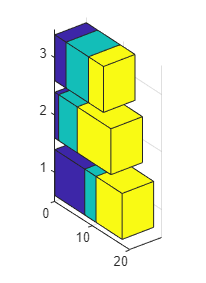Bar color, specified as one of the options in this table.

Color NameShort NameAppearance
`'red'``'r'``'green'``'g'``'blue'``'b'``'cyan'` `'c'``'magenta'``'m'``'yellow'``'y'``'black'``'k'``'white'``'w'`Axes object. If you do not specify an axes object, then `bar3h` creates the bar graph in the current axes.

## Output Arguments

collapse all

Displayed horizontal bars, returned as a vector of `Surface` objects. Use the elements in `h` to access and modify properties of a specific series of horizontal bars after it has been created. The number of `Surface` objects returned depends on the size of `y`. If `y` is a vector, then `h` is one `Surface` object. If `y` is a matrix, then `h` is a vector containing a `Surface` object for each column in `y`.

collapse all

### Series of Bars

A series of bars consists of all the bars that correspond to a specific column in `y`. The `bar3h` function displays each series at a different location along the x-axis. By default, each series is a different color.

In this image, the first series is highlighted.### Group of Bars

A group consists of all the bars at a particular y location.

In this image, the third group is highlighted.## Tips

• You can modify the plotted bars by altering the properties of the returned `Surface` objects. For a list of `Surface` properties, see Surface Properties.

## Version History

Introduced before R2006a

expand all